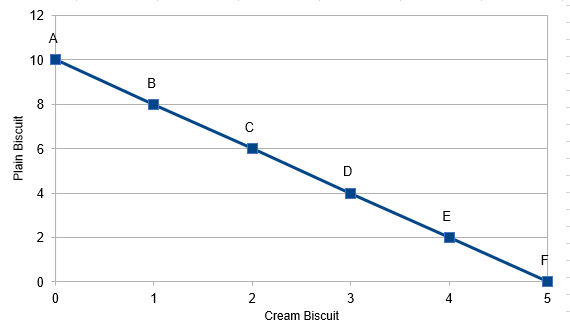# Budget Line: All You Need to Know

## Budget line definition

The budget line, also known as the budget constraint, exhibits all the combinations of two commodities that a customer can manage to afford at the provided market prices and within the particular earning degree.

The budget line is a graphical delineation of all possible combinations of the two commodities that can be bought with provided income and cost so that the price of each of these combinations is equivalent to the monetary earnings of the customer.

It is important to keep in mind that the slope of the budget line is equivalent to the ratio of the cost of two commodities. The slope of the budget constraint possesses distinctive importance.

In other words, the slope of the budget line can be described as a straight line that bends downwards and includes all the potential combinations of the two commodities which a customer can purchase at market value by assigning his/her entire salary. The concept of the budget line is different from the Indifference curve, though both are necessary for consumer equilibrium.

The two basic elements of a budget line are as follows:

• The consumer’s purchasing power (his/her income)
• The market value of both the products

## Equation of a Budget Line

To understand the concept of a budget line in a detailed manner, it is important to understand the mentioned equation. The equation of the budget line equation can be represented as follows:

M = Px × Qx + Py × Qy

Where,

Px is the cost of product X.

Qx is the quantity of product X.

Py is the cost of product Y.

Qy is the quantity of product Y.

M is the consumer’s income.

## Example of a Budget Line

Radha has ₹50 to buy a biscuit. She has a few options to allocate her income so that she receives maximum utility from a limited salary.

 Budget schedule Combination Cream biscuit (@ ₹10 per packet) Plain biscuit (@ ₹5 per packet) Budget allocation A 0 10 10 × 0 + 5 × 10 = 50 B 1 8 10 × 1 + 5 × 8 = 50 C 2 6 10 × 2 + 5 × 6 = 50 D 3 4 10 × 3 + 5 × 4 = 50 E 4 2 10 × 4 + 5 × 2 = 50 F 5 0 10 × 5 + 5 × 0 = 50

To get an appropriate budget line, the budget schedule given can be outlined on a graph.The budget set indicates that the combinations of the two commodities are placed within the affordability margin of a consumer.

Also, read: What is a Budget Set?

## Features of Budget Line

Some of the properties of the budget line are as follows:

Negative slope: If the line is downward, it shows a reverse correlation between the two products.

Straight line: It indicates a continuous market rate of exchange in individual combinations.

Real income line: It denotes the income and the spending size of a customer.

Tangent to indifference curve: It is the point when the indifference curve meets the budget line. This point is known as the consumer’s equilibrium.

You might also want to know about: Economic system in India

## Assumptions of a Budget Line

The budget line is mostly based on the assumption and not reality. However, to get clear and precise results and summary, the economist considers the following points in terms of a budget line:

Two commodities: The economist assumes that the customers spend their income to purchase only two products.

Income of the customers: The income of the customer is limited, and it is designated to buy only two products.

Market price: The cost of each commodity is known to the customer.

Expense is similar to income: It is assumed that the customer spends and consumes the whole income.

## A shift in Budget Line

A budget line includes a consumer’s earnings and the rate of a commodity. These are the two important factors that shift the budget line.

Shift due to change in price: The amount of the product either increases or decreases from time to time. For instance, if the price and income of product A remains constant and the price of product B decreases, then the buying potential of product B automatically increases. Similarly, if the price of B increases and the other factors remain steady, the demand for product B automatically decreases.

Shift due to change in income: Change in income makes a huge difference that leads to a change in the budget line. High income means high purchasing possibility and low income means low purchasing potential, making the budget line to shift.

This concludes the topic, ‘Budget Line’. It is an important concept in economics for students of commerce. To read more about such informative concepts, stay tuned to BYJU’S.

 Important Topics in Economics: